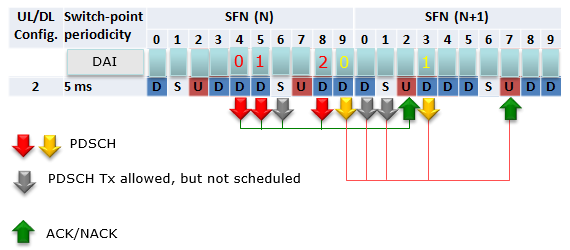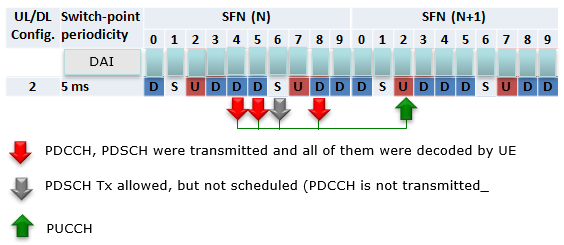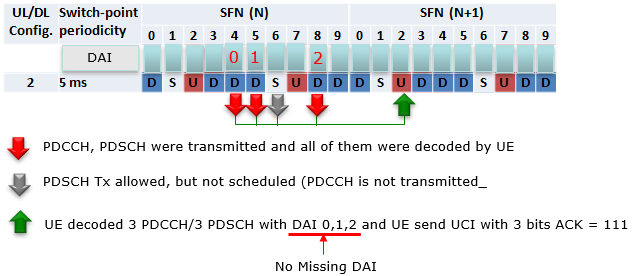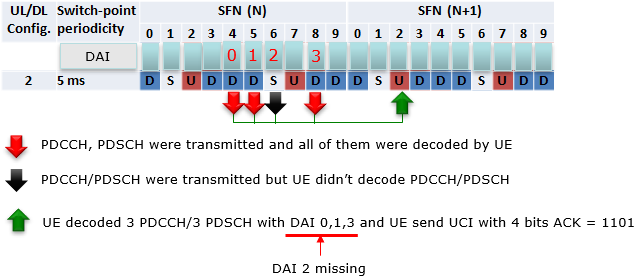4G/LTE - Basic Procedures DAI   DAI stands for Downlink Assignment Index. When you say 'Index', it usually means a unique number assigned to each elements in an array or set. It usually is assigned to each element in increasing order. How DAI is assigned ? Why DAI ?     How DAI is assigned ?   DAI is also a type of index. Then what is the set (or array) to which this index is used for. The set in this case is 'all of the transmitted (scheduled) downlink data(PDSCH) which is bundled to one HARQ ACK/NAC transmission' in TDD operation (If this does not sound clear to you, I would suggest you to read 'HARQ Timing' section first).     Example 01 >   Let's take a look at TDD DL/UL Configuration 2 as an example. Assuming that every DL subframe is scheduled to transmit PDSCH, the DAI is assigned as shown below.Just to make it clearer, I would suggest you to describe this illusteration into words. My version of description is as follows. Subframe 4,5,6,8 in a frame are bundled to subframe 2 in next subframe (Lets call the subframe 4,5,6,8 as Set A) Subframe 9,0,1,3 are bundled to subframe 7 (Lets call the subframe 9,0,1,3 as Set B) All of the subframe listed above are scheduled to transmit PDSCH. When scheduling PDSCH in subframe 4, eNB assigns the index(number) 0 to DAI field of the DCI because this is the first PDSCH transmission in a same set(Set A in this case). When scheduling PDSCH in subframe 5, eNB assigns the index(number) 1 to DAI field of the DCI because this is the second PDSCH transmission in a same set(Set A in this case). When scheduling PDSCH in subframe 6, eNB assigns the index(number) 2 to DAI field of the DCI because this is the third PDSCH transmission in a same set(Set A in this case). When scheduling PDSCH in subframe 8, eNB assigns the index(number) 3 to DAI field of the DCI because this is the fourth PDSCH transmission in a same set(Set A in this case). When scheduling PDSCH in subframe 9, eNB assigns the index(number) 0 to DAI field of the DCI because this is the first PDSCH transmission in a same set(Set B in this case). When scheduling PDSCH in subframe 0, eNB assigns the index(number) 1 to DAI field of the DCI because this is the second PDSCH transmission in a same set(Set B in this case). When scheduling PDSCH in subframe 1, eNB assigns the index(number) 2 to DAI field of the DCI because this is the third PDSCH transmission in a same set(Set B in this case). When scheduling PDSCH in subframe 3, eNB assigns the index(number) 3 to DAI field of the DCI because this is the fourth PDSCH transmission in a same set(Set B in this case).       Example 02 >   Let's take a look at TDD DL/UL Configuration 2 as an example.  This is the same configuration as in Example 01, but with a little bit of different scheduling. In this example, some of the subframe does not transmit(not scheduled) to transmit PDSCH and the DAI is assigned as shown below.Just to make it clearer, I would suggest you to describe this illusteration into words. My version of description is as follows. Subframe 4,5,8 in a frame are bundled to subframe 2 in next subframe (Lets call the subframe 4,5,8 as Set A) Subframe 9,3 are bundled to subframe 7 (Lets call the subframe 9,3 as Set B) All of the subframe listed above are scheduled to transmit PDSCH. When scheduling PDSCH in subframe 4, eNB assigns the index(number) 0 to DAI field of the DCI because this is the first PDSCH transmission in a same set(Set A in this case). When scheduling PDSCH in subframe 5, eNB assigns the index(number) 1 to DAI field of the DCI because this is the second PDSCH transmission in a same set(Set A in this case). subframe 6 is NOT secheduled for PDSCH. When scheduling PDSCH in subframe 8, eNB assigns the index(number) 2 to DAI field of the DCI because this is the fourth PDSCH transmission in a same set(Set A in this case). When scheduling PDSCH in subframe 9, eNB assigns the index(number) 0 to DAI field of the DCI because this is the first PDSCH transmission in a same set(Set B in this case). subframe 0 is NOT secheduled for PDSCH.. subframe 1 is NOT secheduled for PDSCH. When scheduling PDSCH in subframe 3, eNB assigns the index(number) 1 to DAI field of the DCI because this is the the second PDSCH transmission in a same set(Set B in this case).       Why DAI ?   As you see in the example shown above or shown in this not  in more details, the number of Ack/Nack bits in PUCCH varies depending on Uplink/Downlink configuration and the real PDSCH scheduling within the pattern. If we assume that eNB always schedule PDSCH for every possible DL subframe (including special subframe) and all of the PDCCH (DCI) is successfully recieved and decoded by UE, the number of ACK/Nack Bits (Ack/Nack bit length) can be determined directly from Uplink/Downlink configuration, however in reality eNB does not always schedule PDSCH at every subframe and we cannot guarantee that UE would be able to successfully decode the PDCCH (DCI). In this case, how the UE can figure out the Ack/Nack Bit length ?   Let's think of a case shown below as an example and assume all the PDSCH is single codeword.Now let's assume that UE is trying to construct a UCI at SFN(N+1), subframe 2. According to the diagram above and assume that UE has successully decoded PDCCH and PDSCH transmitted from SFN(N), subframe 4,5,8 but didn't received any PDCCH/PDSCH at SFN(N), Subframe 6.  Which Ack/Nack UCI the UE should send at SFN(N+1), subframe 2 ? Should it be '111' ? or '1101' ?  If UE knows, eNB scheduled PDCCH only at  SFN(N), subframe 4,5,8 (i.e, skipping SFN(N), subframe 6), then the UCI should be '111'. If UE knows, eNB scheduled PDCCH at  SFN(N), subframe 4,5,6,8 and it failed to decode PDCCH at subframe 6. The UCI should be '1101'. However the problem is 'UE cannot figure out whether eNB sent PDCCH at subframe 6 or not'. Then how UE can construct the proper UCI in this case ? That's where DAI kicks in. DAI let UE figure out whether it recieved all the scheduled PDCCH/PDSCH or missed any PDCCH. To be honest, I don't think my written explanation here would be clear to you. Would following diagram would be any more help ? I hope it sounds clearer.  If you can clearly understand the difference between case 1 and 2, it was successful for me to understand the basic concept/role of DAI.   Case 1 >Case 2 >